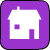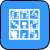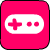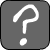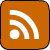Mathematical Views The image to the right is showing what you would see if you were sitting at the point origin and looking down at spheres on a logarithmic curve (specifically y = -ln(x)) at every integral value of x. This is probably easier to understand with a diagram:You are at the point shown in blue looking out at the grey balls which are all on the curve of the type and with the equation above. If you would like to suggest some other functions to feature, comment on my blog or reddit. Change the function displayed: logarithm quadratic linear hyperbola sine cosineRemember me Login details will work on bothPurple Stereo CountdownandCats in Drag.
Hide